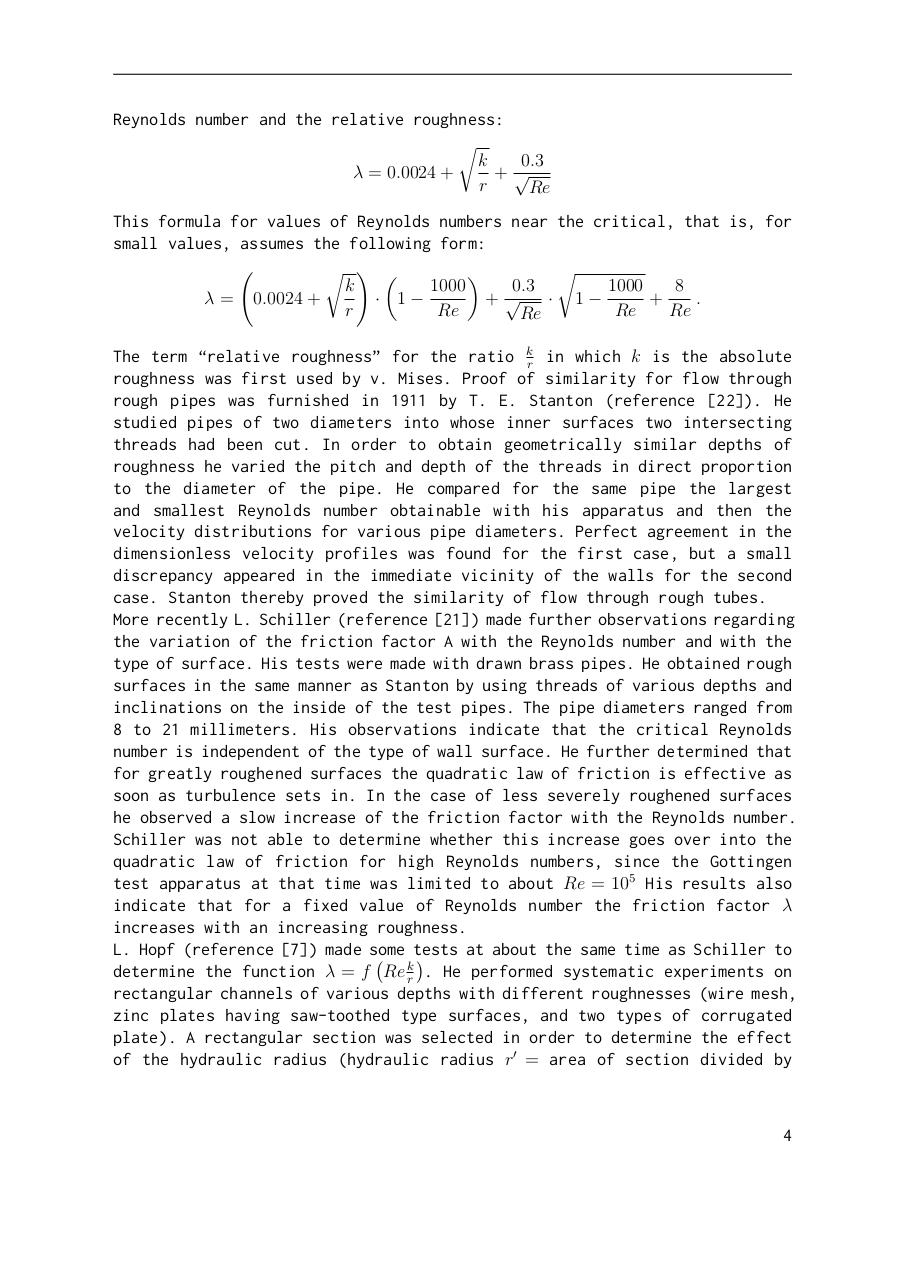# Johann Nikuradse. LAWS OF FLOW IN ROUGH PIPES.pdfPage 1 2 3 45664

#### Text preview

Reynolds number and the relative roughness:
r
k
0.3
λ = 0.0024 +
+√
r
Re
This formula for values of Reynolds numbers near the critical, that is, for
small values, assumes the following form:
r ! 
r

k
1000
0.3
1000
8
· 1−
+√
· 1−
+
.
λ = 0.0024 +
r
Re
Re
Re
Re
The term “relative roughness” for the ratio kr in which k is the absolute
roughness was first used by v. Mises. Proof of similarity for flow through
rough pipes was furnished in 1911 by T. E. Stanton (reference ). He
studied pipes of two diameters into whose inner surfaces two intersecting
threads had been cut. In order to obtain geometrically similar depths of
roughness he varied the pitch and depth of the threads in direct proportion
to the diameter of the pipe. He compared for the same pipe the largest
and smallest Reynolds number obtainable with his apparatus and then the
velocity distributions for various pipe diameters. Perfect agreement in the
dimensionless velocity profiles was found for the first case, but a small
discrepancy appeared in the immediate vicinity of the walls for the second
case. Stanton thereby proved the similarity of flow through rough tubes.
More recently L. Schiller (reference ) made further observations regarding
the variation of the friction factor A with the Reynolds number and with the
type of surface. His tests were made with drawn brass pipes. He obtained rough
surfaces in the same manner as Stanton by using threads of various depths and
inclinations on the inside of the test pipes. The pipe diameters ranged from
8 to 21 millimeters. His observations indicate that the critical Reynolds
number is independent of the type of wall surface. He further determined that
for greatly roughened surfaces the quadratic law of friction is effective as
soon as turbulence sets in. In the case of less severely roughened surfaces
he observed a slow increase of the friction factor with the Reynolds number.
Schiller was not able to determine whether this increase goes over into the
quadratic law of friction for high Reynolds numbers, since the Gottingen
test apparatus at that time was limited to about Re = 105 His results also
indicate that for a fixed value of Reynolds number the friction factor λ
increases with an increasing roughness.
L. Hopf (reference ) made some tests
at about the same time as Schiller to

k
determine the function λ = f Re r . He performed systematic experiments on
rectangular channels of various depths with different roughnesses (wire mesh,
zinc plates having saw-toothed type surfaces, and two types of corrugated
plate). A rectangular section was selected in order to determine the effect
of the hydraulic radius (hydraulic radius r0 = area of section divided by

4